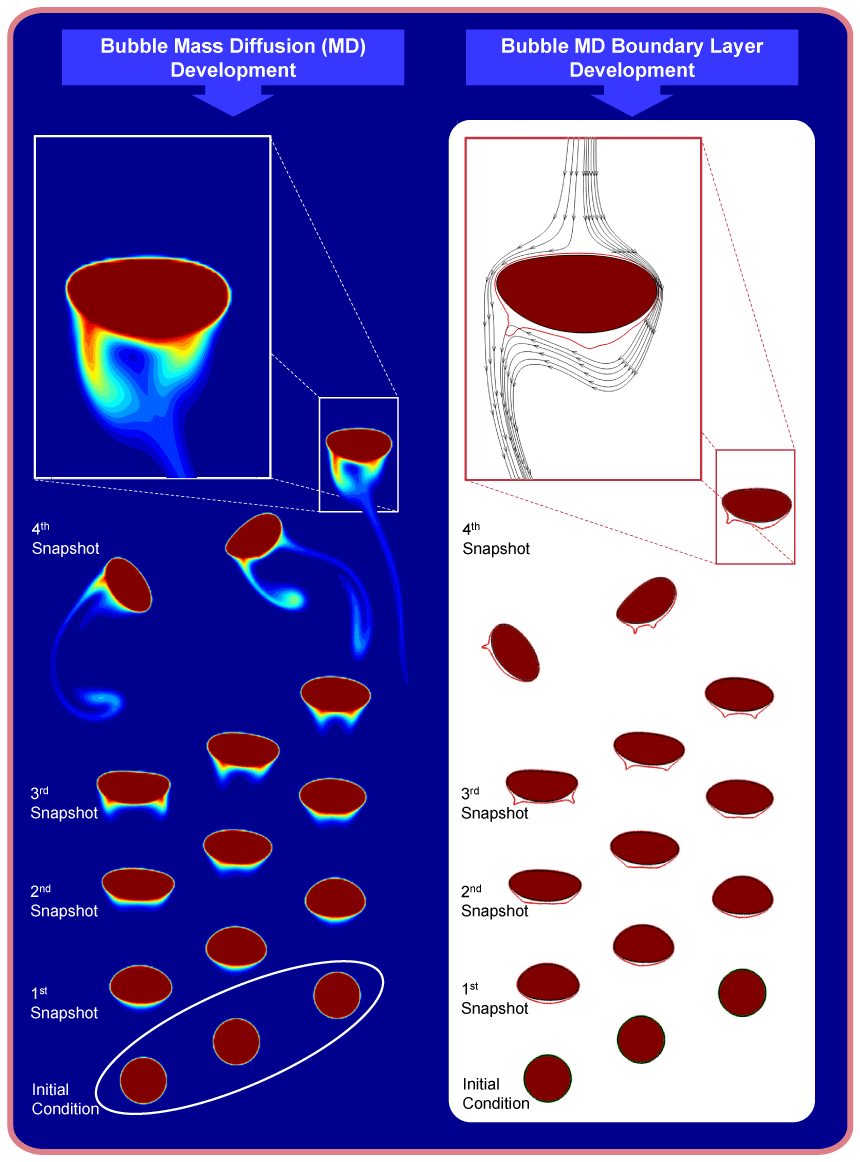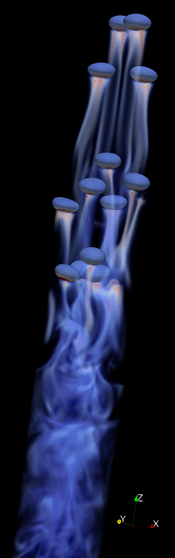Multiscale Simulation of Mass Transfer in Bubbly FlowBubble column is one of the widely used processing equipments in chemical and petrochemical industry. Its advantage is its simple structure which results in lower production cost and maintenance. Bubble column generally consists of a cylinder full of liquid (as shown on the right) with gas injected from the bottom of the column. The gas bubbles start rising because of buoyancy and as they rise, gas diffuses into the surrounding liquid and reacts with the dissolved reactants. The products of these reactions are generally very dependent on the flow characteristics, including gas bubbles behavior, and bubble column design. Large amount of chemicals is processed in these columns every year and small improvement in their performance will lead to a significant amount of savings. In spite of bubble column's simple design, prediction of its behavior is challenging due to the nonlinear interactions of rising bubbles. Nowadays, we still use small scale laboratory testing and semi-empirical or empirical correlations for the design of these columns. With the growth of Computational Fluid Dynamics (CFD) in different engineering applications, it is beneficial to use this valuable tool to improve the design of bubble columns.

Computational Fluid Dynamics have considerably grown in the last three decades and we are now able to simulate large calculations of fluid flow in a wide range of applications. However, still when we need to include additional physics to the problem, usually the disparity between length and time scales of different physics in the problem exponentially increases the computational costs. For example, mass transfer from gas bubbles into surrounding liquid generally takes place on a smaller length and time scale than the flow length and time scale, which results in very thin mass boundary layer next to the bubble interface. In order to correctly calculate the mass transfer from the gas bubble, one needs to resolve this thin mass boundary layer, which either by refining the computational grid globally or locally (Adaptive Mesh Refinement), results in a considerable increase of computational cost.Here, we use a Front Tracking method to follow the interface. In order to resolve the thin mass film next to the interface, a mass boundary layer equation is derived which is solved on the interface points (as shown in the left figure, red dots). Using this equation, the evolution of the mass boundary layer is followed and whenever the boundary layer thickness is grown over a predefined boundary layer limit, mass is transferred using a source term from the boundary layer to the grid points next to the bubble. The transferred mass is then advected and diffused in the rest of the domain by solving an advection-diffusion equation on the Cartesian grid.

In the figure shown below, I demonstrate the 2D simulation of three rising buoyant bubbles in an initially stagnant flow. Concentration inside the bubbles is set to a constant value throughout the simulation and the surrounding fluid is set to have zero concentration initially. Here, I show 5 snapshot of the simulation. On the left column the mass concentration is shown while on the right column boundary layer thickness is calculated and shown using our model by red lines surrounding the bubbles. More detail on our model, 2D implementation and verification of our model can be found here.We also implemented our subscale model in a 3D front tracking code. The model is tested and validated by directly comparing our results with experimental results. We have been able to simulate dissolution CO2 bubbles in the glycerol-water solution, with a high Schmidt number of 8260 and a Reynolds number of about 300 with minimal computational cost for the mass transfer. Based on the minimum thickness of mass boundary layer we estimate that computational cost is reduced at least by two order of magnitude. More details on the comparison with the experimental results and correlations from the literature is published in this journal article.
In the following fluid dynamics video, a simulation of the mass transfer from buoyant bubbles is demostrated, which is done using a Front Tracking method for the tracking of interface and our subscale description for the transfer of mass from the bubble into the domain. This video has been submitted in the gallery of fluid motion, APS 2012 San Diego, and also could be found at this address in arXiv. This simulation shows 13 moving bubbles in a periodic domain, 3db X 3db X 48db, where db is the bubble diameter. The grid resolution is 64 X 64 X 1024, which results in about 21 cell across one bubble diameter. The flow non-dimensional governing parameters are Eo = 2.81 and Mo = 4.5 * 10^-7 with density and viscosity ratio of 0.1 and for the mass transfer we have Sc = 60. In the movie, bubbles' surfaces are colored to show the mass boundary layer thickness, with blue showing a close to zero value and red showing the maximum value. Mass concentration inside the domain is colored from transparent blue for low value, 0, to solid red for high value, 1. Time is non-dimensionalized with sqrt(db/g). A snapshot of the simulation is shown on the right hand side.